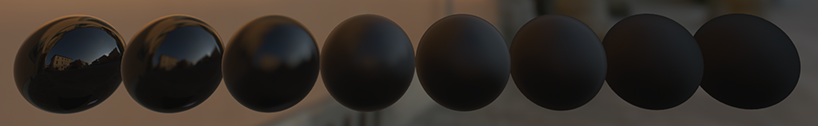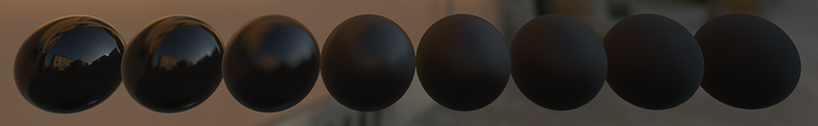September 10, 2014

# Physically Based Shading on Mobile

By Brian Karis

This post is the first in hopefully a series of in depth, highly technical discussions of features in UE4.

This is a follow up to my talk Real Shading in Unreal Engine 4. That talk explained the shading model used in UE4. This post assumes the reader is familiar with the course notes.

There have been a few minor changes since that presentation. I removed Disney's "hotness" modification to the geometry term. With Eric Heitz's recent work I may be improving this again to add a correlation between the shadowing and masking. The Cavity parameter never made it in. We were poised to make that change right before I presented but have found uses for controllable dielectric specular reflectance. Instead we use the Specular parameter as defined in Disney's model.

What I really want to talk about is how this shading model was adapted for mobile platforms. For a great run down of UE4's mobile renderer check out these slides from GDC. For some examples of its use check out the Zen tech demo which was running 4xMSAA 1080p at 30hz on an iPad Air or the Soul tech demo.

A major goal from the beginning was that we wanted the same content creation workflow as high end PC. We also wanted to support rendering the same assets so that when running on a mobile device, everything looks as close as we can to the high end renderer. This means many tiny things like getting the gamma right but it also means shading needs to work in the same way so that a material appears to behave the same on PC, consoles and mobile devices. To do that, we support the same material model I explained in my talk. BaseColor, Metallic, Specular, Roughness. These parameters operate in the exact same way.

Obviously there is far less compute power on mobile devices so we can't evaluate the BRDF for every light. In fact, we only support a single directional sun light that is calculated dynamically. The shadows are precomputed and stored as signed distance fields in texture space. All other lights are baked into lightmaps. The lightmaps use a similar HDR encoded SH directional representation as high end but adapted to better compress with PVRTC which doesn't have separate, uncorrelated, alpha compression. Like high end, precomputed specular uses preconvolved environment maps. The exact same preconvolution is used and they are similarly normalized and scaled by the lightmap. For mobile, only one environment map is fetched per pixel.

#### Environment BRDF

The interesting bit comes from how we calculate Environment BRDF which for high end is precomputed with Monte Carlo integration and stored in a 2D LUT. Dependent texture fetches are really expensive on some mobile hardware but even worse is the extremely limiting 8 sampler limit of OpenGL ES2. Chewing up a sampler with this LUT was totally unacceptable. Instead, I made an approximate analytic version based on Dimitar Lazarov's work. The form is similar but adapted to fit our shading model and roughness parameter. The function is listed below:

half3 EnvBRDFApprox( half3 SpecularColor, half Roughness, half NoV )
{
const half4 c0 = { -1, -0.0275, -0.572, 0.022 };
const half4 c1 = { 1, 0.0425, 1.04, -0.04 };
half4 r = Roughness * c0 + c1;
half a004 = min( r.x * r.x, exp2( -9.28 * NoV ) ) * r.x + r.y;
half2 AB = half2( -1.04, 1.04 ) * a004 + r.zw;
return SpecularColor * AB.x + AB.y;
}


This means we have plausible Fresnel and roughness behavior for image based lighting.##### EnvBRDF used on high end##### EnvBRDFApprox used on mobile

We detect when Metallic and Specular material outputs aren't connected (meaning the defaults are used). This is true for a typical nonmetal. In this case we can further optimize the function.

half EnvBRDFApproxNonmetal( half Roughness, half NoV )
{
// Same as EnvBRDFApprox( 0.04, Roughness, NoV )
const half2 c0 = { -1, -0.0275 };
const half2 c1 = { 1, 0.0425 };
half2 r = Roughness * c0 + c1;
return min( r.x * r.x, exp2( -9.28 * NoV ) ) * r.x + r.y;
}


A similar optimization is made to avoid the environment map altogether. Some games optimize for no specular but that isn't energy conserving. We instead have a "Fully rough" flag which sets Roughness=1 and optimizes out constant factors. We can see that:

EnvBRDFApprox( SpecularColor, 1, 1 ) == SpecularColor * 0.4524 - 0.0024


From there I make the simplification:

DiffuseColor += SpecularColor * 0.45;
SpecularColor = 0;


We only use this flag on select objects that really need the extra performance.

#### Directional Light

The last bit I want to touch on is how the sun light is calculated. I mentioned earlier that the sun is the only light computed dynamically. Unfortunately, even for a single light the full high end shading model is still too heavy. We have just calculated a portion of it in an approximate preintegrated form with the EnvBRDF though. We also already have the reflection vector which was used to sample the environment map. The idea is to analytically evaluate the radially symmetric lobe that is used to prefiler the environment map and then multiply the result with EnvBRDF just like we do for IBL. Think of this as analytically integrating the lobe against the incoming light direction instead of numerically integrating like we do with the environment map.

First, we replace the GGX NDF with Blinn. Blinn is then approximated with a radially symmetric Phong lobe.

$$\newcommand{\nv}{\mathbf{n}} \newcommand{\lv}{\mathbf{l}} \newcommand{\vv}{\mathbf{v}} \newcommand{\hv}{\mathbf{h}} \newcommand{\mv}{\mathbf{m}} \newcommand{\rv}{\mathbf{r}} \newcommand{\ndotl}{\nv\cdot\lv} \newcommand{\ndotv}{\nv\cdot\vv} \newcommand{\ndoth}{\nv\cdot\hv} \newcommand{\ndotm}{\nv\cdot\mv} \newcommand{\vdoth}{\vv\cdot\hv} D_{Blinn}(\hv) = \frac{1}{ \pi \alpha^2 } (\ndoth)^{ \left( \frac{2}{ \alpha^2 } - 2 \right) } \approx \frac{1}{ \pi \alpha^2 } (\rv\cdot\lv)^{ \left( \frac{1}{ 2 \alpha^2 } - \frac{1}{2} \right) }$$

Where $\rv$ is the reflection direction $\rv = 2(\ndotv)\nv - \vv$

Phong is further approximated with a Spherical Gaussian:

$$x^n \approx e^{ (n + 0.775) (x - 1) }$$

This brings us to the final optimized form:

half D_Approx( half Roughness, half RoL )
{
half a = Roughness * Roughness;
half a2 = a * a;
float rcp_a2 = rcp(a2);
// 0.5 / ln(2), 0.275 / ln(2)
half c = 0.72134752 * rcp_a2 + 0.39674113;
return rcp_a2 * exp2( c * RoL - c );
}


Where $\frac{1}{\pi}$ is factored into the light's color.

In the end we have a very cheap but reasonable shading model. Specular is energy conserving, behaves sensibly at grazing angles, and works with both analytic and image based light sources. Not even mobile hardware is an excuse to not to be physically based anymore.

Epic Games, Inc. gives you permission to use, copy, modify, and distribute the code samples in this article as you see fit with no attribution required.